## 1.3.1 The NOPO data structure

The internal representation of a mesh must allows us to find a particular value (quantity stored in the representation) rapidly, or to calculate a value easily from information presented (quantity deduced from stored information). A mesh is therefore, in general, an assembly of values and arrays of values containing the set of geometric elements which constitute it. The following information is necessary for each element:

• the nature of the element: segment, triangle, quadrangle, tetrahedra, pentahedra, hexahedra, or a particular element;
• the list of the vertices of the element;
• the element connectivity and topology (the connectivity describes the connectivity between the vertices, the topology defines the edges and faces of the elements in relation to the vertices);
• the number and list of the nodes;
• the coordinates of the vertices;
• the physical attributes of the element:
• a sub-domain number or material number. This number, assigned to each element, characterizes it with regard to a treatment of type:

 sub-domain number"volumetric" treatment

• reference numbers or references. This number is assigned to the items which constitute an element (faces, edges, points) and enables these items to be characterized with regard to a treatment of type:

 reference number"sub-volumetric" treatment

The MODULEF code stores this information in a NOPO data structure, constituting the natural interface between the different mesh modules.

Remark:

• The sub-domains : The notion of materials is refined to distinguish two identical media subject to different conditions: as many sub-domain numbers are assigned as required to make this distinction;
• The references : This attribute has two functions:
• to characterize the items (edges and points) with respect to a boundary condition type treatment (loads, prescribed values, etc.)
• to describe a boundary from a geometric point of view: i.e. to associate a curve equation to a portion of the boundary and force, in this way, the mesh to approach this curve.

As many reference numbers are assigned as required in order to incorporate the entire set of descriptions.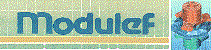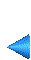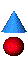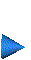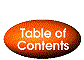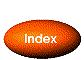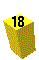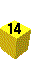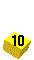Next: 1.3.2 Introduction of a curve Up: 1.3 Main ideas Prev: 1.3 Main ideas Index Contents